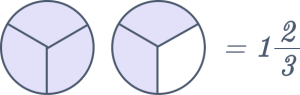# Fraction Calculator

The fraction calculator by The Mathematics Master is used to add, subtract, multiply, and divide fractions. Answers are expressed as the lowest terms of fractions or as reduced forms of mixed numbers.

$$\dfrac{a}{b} + \dfrac{c}{d} = ?$$

This fraction calculator can work with expressions containing fractions, integers, decimals, mixed numbers, and simple fraction operations. In addition, the calculator helps derive values from various fractional operations.

## What is a Fraction?

A fraction is a number that, in mathematics, represents a portion of a whole. There are two parts: a numerator and a denominator. The denominator is the total number of pieces that make up the whole, while the numerator is the number of equally sized portions of the whole. For instance, the numerator of the fraction 3/8 is 3, and the denominator is 8. An illustration that is more concrete would be a pie with eight slices. A fraction would have 1 of those eight pieces as the numerator and the remaining eight slices, which make up the entire pie, as the denominator. The fraction of the pie remaining after five slices would be 3/8. Remember that a fraction’s denominator cannot be zero because it would render the fraction meaningless.

$$\dfrac{a}{b} + \dfrac{c}{d} = \dfrac{ad+bc}{bd}$$

$$\dfrac{1}{3} + \dfrac{1}{4} = \dfrac{(1 × 4) + (1 × 3)}{4 × 3} = \dfrac{7}{12} = 0.58333$$

## Subtracting fractions example

$$\dfrac{a}{b} – \dfrac{c}{d} = \dfrac{ad – bc}{bd}$$

$$\dfrac{1}{5} – \dfrac{1}{8} = \dfrac{(1 × 8) – (1 × 5)}{5 × 8} = \dfrac{3}{40} = 0.075$$

## Multiplying fractions example

$$\dfrac{a}{b} . \dfrac{c}{d} = \dfrac{ac}{bd}$$

$$\dfrac{1}{3} × \dfrac{1}{4} = \dfrac{1 × 1}{3 × 4} = \dfrac{1}{12}$$

## Dividing fractions example

$$\dfrac{\frac{a}{b}}{\frac{c}{d}} = \dfrac{ad}{bc}$$

$$\dfrac{1}{2} ÷ \dfrac{1}{3} = \dfrac{1}{2} × \dfrac{3}{1} = \dfrac{1 × 3}{2 × 1} = \dfrac{3}{2} = 1\dfrac{1}{2}$$

## FAQs

How do you write the fraction 4/22 as a decimal?

Step-by-step explanation:

x = 4/22 = 0.1818

Alternatively, you can use the fraction to decimal calculator.

How to convert fractions to mixed numbers?

5/3 = 5 ÷ 3 = 1 R2 OR 1 ⅔We can also use an online free mixed faction calculator to convert fractions to mixed numbers.What is a partial fraction decomposition calculator?

A partial fraction in mathematics is a way to represent a rational function (the product of two polynomials) as the total of smaller rational expressions. The numerator and denominator of a function are entered into the partial fraction decomposition calculator to decompose the function.